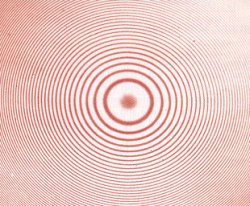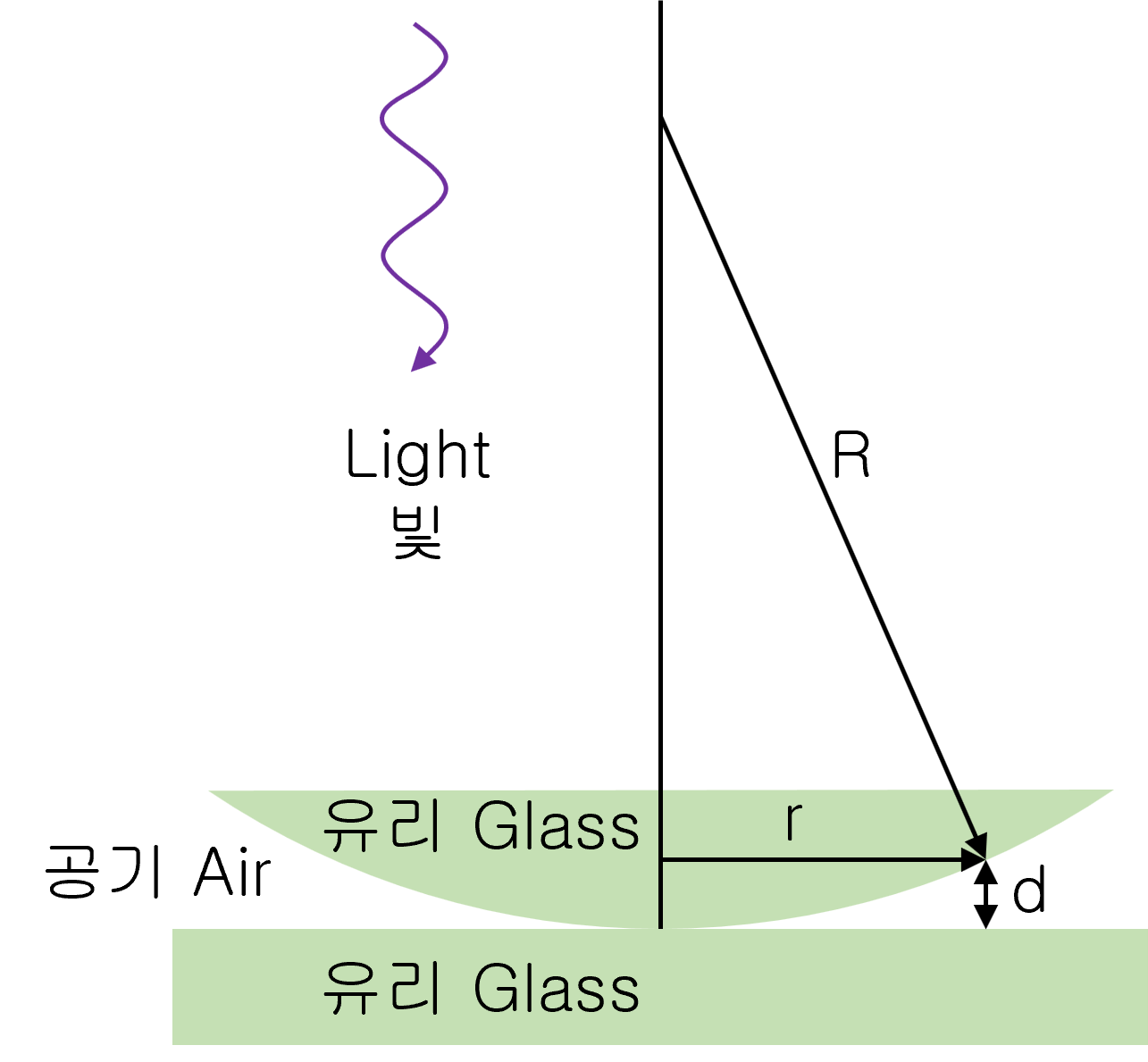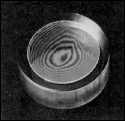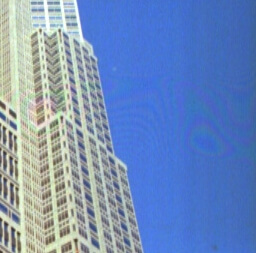# Newton Ring

## The Newton ring

The interference of two reflections causes Newton rings.

We can observe the Newton Rings when light is reflected from a plano-convex lens of a long focal length placed in contact with a plane glass plate. A thin air film is formed between the plate and the lens. The thickness of the air film varies. If the lens plate system is illuminated with monochromatic light falling on it, concentric bright and dark interference rings are observed in reflected light. These circular fringes were discovered by Newton and are called Newton’s rings.## Newton ring calculation formula

If the wavelength of the incident light is λ, the radius of curvature of the lens is R, and the distance from the lens center is r, the light intensity I is expressed as follows.$I\, =\, 4{ I }_{ 0 }{ Sin }^{ 2 }(\frac { \pi { r }^{ 2 } }{ \lambda R } )$

## Applications of the Newton Ring Effect

• It is applied to check whether the curved surface of the lens is uniform.• The Newton ring phenomenon can occur when film images are read through a scanner.
This is a phenomenon because the film does not exactly touch the surface of the scanner and is slightly raised.
You can prevent this by loading the film so that it is in direct contact with the scanner’s glass.
(EPSON Color Guide)